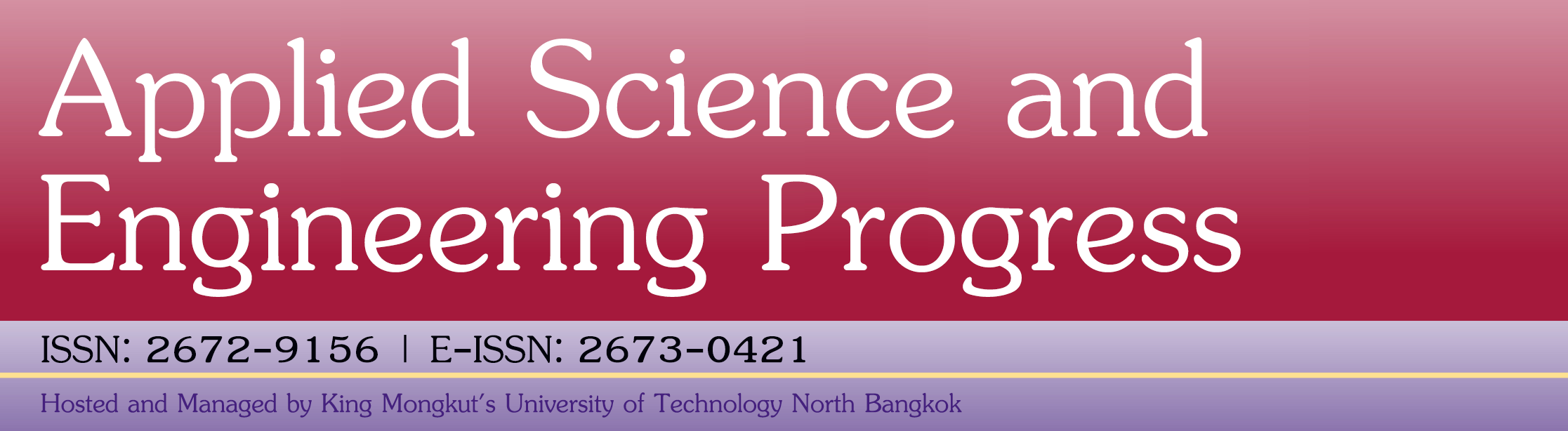#### Hydrolysis Optimization of Isoflavone Conversion in Soy Germ (Chiang Mai 60) Using Response Surface Methodology (RSM)

Sriwiang Tipkanon, Ratchanee Charoen, Wanticha Savedboworn, Ahmed Moursy Abdallah

#### Abstract

Response surface methodology (RSM) was employed to optimize the hydrolysis conditions of isoflavone conversion in soy germ by using central composite design (CCD) of three factors. A five-level, three variables design was adopted. The three independent variables investigated in this experiment were pH (X1) 3 – 8; reaction temperature (X2) 25 – 75 °C; and reaction time (X3) 2 – 12 h. Dependent variables were highly fitted to the regression equation for the both of total amount of isoflavone glucosides (Y1) and total amount of isoflavone aglycones (Y2). The high regression coefficient values indicated that the variables were highly fitted to the regression equation for the both of total isoflavone glucosides (R2 = 0.937) and total isoflavone aglycones (R2 = 0.956) concentration. Optimum hydrolysis conditions for maximizing the determination of total isoflavone aglycones content were: pH = 5.3, reaction temperature = 51 °C and reaction time = 7.5 h.

Full Text: PDF

### Refbacks

• There are currently no refbacks.